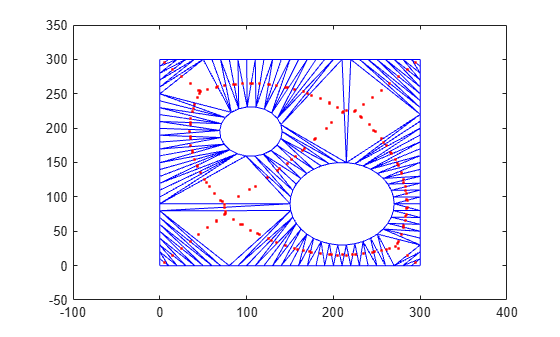circumcenter

Circumcenter of triangle or tetrahedron

Description

example

C = circumcenter(TR) returns the coordinates of the circumcenters for each triangle or tetrahedron in the triangulation TR.

example

C = circumcenter(TR,ID) returns the coordinates of the circumcenters for the triangles or tetrahedra indexed by ID. The identification numbers of the triangles or tetrahedra in TR are the corresponding row numbers of the property TR.ConnectivityList.

example

[C,r] = circumcenter(___) also returns the radii of the circumscribed circles or spheres in TR for either of the previous syntaxes.

Examples

collapse all

Load 2-D triangulation data and create a triangulation representation.

TR = triangulation(tri,x,y);

Compute the circumcenters of each triangle in TR.

C = circumcenter(TR);

Plot the triangulation along with the circumcenters in red. The $x$-coordinates of the circumcenters are contained in the first column of C and the corresponding $y$-coordinates are contained in the second column.

triplot(TR)
axis([-100 400 -50 350])
hold on
plot(C(:,1),C(:,2),'r.')
hold offCreate a Delaunay triangulation for a set of points.

rng default;
P = rand(10,3);
TR = delaunayTriangulation(P);

Compute the circumcenters of the first five tetrahedra in TR, and the radii of their circumscribed spheres.

[C,r] = circumcenter(TR,[1:5]')
C = 5×3

13.2189   -2.3004   -0.4970
-0.4884    0.6741   -0.1140
0.3089    0.1067   -0.3349
0.2805    0.7532    0.5855
0.8514    2.1609    0.9824

r = 5×1

12.6978
1.1775
1.1135
0.3670
1.4112

Input Arguments

collapse all

Triangulation representation, specified as a scalar triangulation or delaunayTriangulation object.

Data Types: triangulation | delaunayTriangulation

Triangle or tetrahedron identification, specified as a scalar or a column vector whose elements each correspond to a single triangle or tetrahedron in the triangulation object. The identification number of each triangle or tetrahedron is the corresponding row number of the ConnectivityList property.

Data Types: double

Output Arguments

collapse all

Circumcenters of triangles or tetrahedra, returned as a two-column matrix for 2-D coordinates or a three-column matrix for 3-D coordinates.

Data Types: double

Radii of the circumscribed circles or spheres, returned as a scalar or vector.

Data Types: double